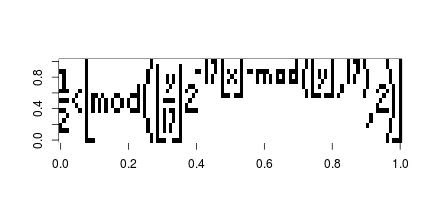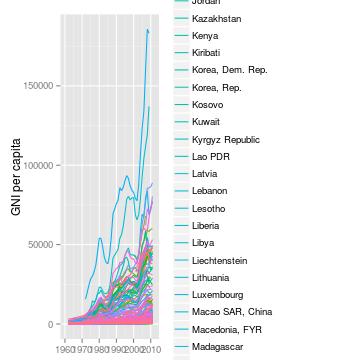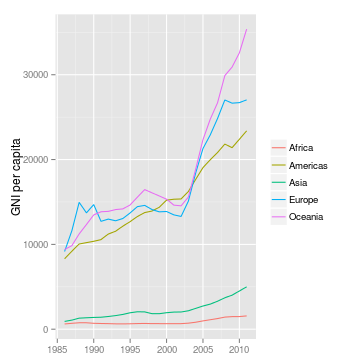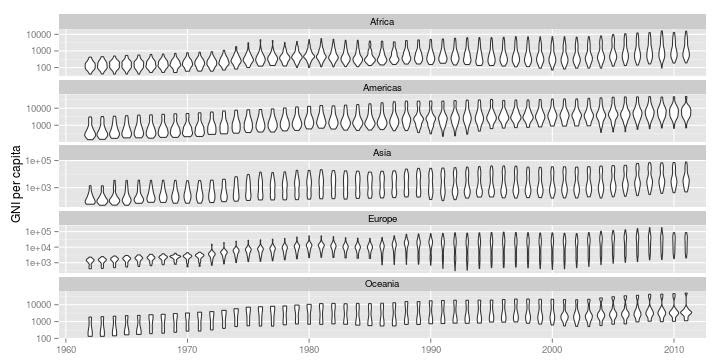## Sunday, March 24, 2013

### Tupper's self-referential formula

Can't remember where I first came across this equation but the Tupper's self referential equation, is a very interesting formula that when graphed in two dimension plane it reproduces the formula.

$\frac{1}{2} < \left\lfloor \bmod\left(\left\lfloor\frac{y}{17}\right\rfloor2^{-17\lfloor x\rfloor - \bmod(\lfloor y \rfloor, 17)}, 2\right)\right\rfloor$

I first thought this would be a quick 5 min exercise which turned into a 3 hour work, the obstacle was that the constant “k” used in the formula is an extremely big integer and can not be handled in R naturally.

After a little search Large integer in R and play around it seems that the gmp library seems to work well.

First you will need to install GMP (The GNU Multiple Precision Arithmetic Library) from http://gmplib.org/, then install the gmp library within R.

## Load the library after installing GMP
library(gmp)

## Define the constant k
k = as.bigz("960939379918958884971672962127852754715004339660129306651505519271702802395266424689642842174350718121267153782770623355993237280874144307891325963941337723487857735749823926629715517173716995165232890538221612403238855866184013235585136048828693337902491454229288667081096184496091705183454067827731551705405381627380967602565625016981482083418783163849115590225610003652351370343874461848378737238198224849863465033159410054974700593138339226497249461751545728366702369745461014655997933798537483143786841806593422227898388722980000748404719")

## The tupper's formula
tupper = function(x, y, k){
z1 = as.bigz(y + k)
z2 = as.bigq(z1/17)
z3 = 2^(-17 * x - as.bigz(z1%%17))
0.5 < floor(as.bigz(z2 * z3)%%2)
}

## The x and y axis
x = 0:105
y = 0:16

## Compute the matrix
a = matrix(0, nc = length(x), nr = length(y))
for(i in seq_along(x)){
a[ ,107 - i] = rev(tupper(x[i], y, k = k))
}


Here is the plot

image(t(a), col = c("white", "black"))## Wednesday, March 20, 2013

### Violin plots and regional income distribution

While preparing my slides for statistical graphics, a plot really caught my eye when I was playing around with the data.

I started off by plotting the time seriesof GNI per capita by country, and as expected it got quite messy and incomprehensible.

## Download and manipulate the data
library(FAOSTAT)
raw.lst = getWDItoSYB(indicator = c("NY.GNP.PCAP.CD", "SP.POP.TOTL"))
raw.df = raw.lst[["entity"]]
traw.df = translateCountryCode(raw.df, from = "ISO2_WB_CODE", to = "UN_CODE")
mraw.df = merge(traw.df, FAOregionProfile[, c("UN_CODE", "UNSD_MACRO_REG")])
final.df = mraw.df[!is.na(mraw.df$UNSD_MACRO_REG), ] ## Simple ugly time series plot ggplot(data = final.df, aes(x = Year, y = NY.GNP.PCAP.CD)) + geom_line(aes(col = Country)) + labs(x = NULL, y = "GNI per capita")So I decided to compute the weighted average by region to examine the regional trends. ## Compute regional aggregates based on UN M49 definition reg.df = aggRegion(aggVar = "NY.GNP.PCAP.CD", weightVar = "SP.POP.TOTL", data = traw.df, keepUnspecified = FALSE, aggMethod = "weighted.mean", relationDF = data.frame(UN_CODE = FAOregionProfile[, "UN_CODE"], REG_NAME = FAOregionProfile[, "UNSD_MACRO_REG"])) ## Plot regional aggregates ggplot(data = reg.df[!is.na(reg.df$NY.GNP.PCAP.CD), ],
aes(x = Year, y = NY.GNP.PCAP.CD)) +
geom_line(aes(col = REG_NAME)) +
labs(x = NULL, y = "GNI per capita", col = "")I can now see the trend clearly, but there are two problems with this approach. First, the variability within region is vast and thus the weighted average or any summary statistic such as quantile can be misleading and it does not tell me what is going on within the regions. Secondly, since a minimum of 65% of the country must be present in order to compute the aggregation, no statistics was available prior to 1985.

While I was carrying out regional comparisons with box-plot and violin plot I thought why not plot them accross time as well! So here is the final graph:

## Time series violin plot
ggplot(data = final.df,
aes(x = as.character(Year), y = NY.GNP.PCAP.CD)) +
geom_violin() + scale_y_log10() +
facet_wrap(~UNSD_MACRO_REG, ncol = 1, scales = "free_y") +
scale_x_discrete(breaks = as.character((seq(1960, 2010, by = 10))),
labels = as.character((seq(1960, 2010, by = 10)))) +
labs(x = NULL, y = "GNI per capita")Now I can compare the regions, but at the same time I can see the within region income distribution. It amazes me how the income distribution diverges in Europe and Oceania while America and Asia moves towards a bell shaped distribution. Growth in Africa appears to be slow, but there are several countries which are growing at a faster rate and pushing the tail of the distribution. Although some of the variability in the density may have resulted from independence of countries, nonetheless it is still infromative.